## Saturday, August 24, 2013

### Motivation

Basic linear regression in R is super easy. Just use the lm() function, summarize the model with summary() and you're done. But as researchers we need more than that. What if our model is more complicated, like if our errors are heteroskedastic? And once we have run our regressions with our adjusted standard errors, how do we export the results for presentation in word or latex documents? This post will go over exactly these things, with the help of the stargazer package created by my fellow Harvard grad student Marek Hlavac. More posts about stargazer can be found here on this blog.

Today we are going to analyze how temperature affects ice cream sales. We just make ourselves a little dataset like so:

set.seed(5)
temp <- rnorm(500, mean=80, sd=12)
sales <- 2+temp*3

for(i in 1:length(sales)){
if(temp[i]<75 | temp[i]>95) sales[i] <- sales[i]+rnorm(1,0,25 )
else sales[i] <- sales[i]+rnorm(1,0,8 )
}

female <- rnorm(500, mean=.5, sd=.01)
icecream <- as.data.frame(cbind(temp, sales, female))

head(icecream)

##     temp sales female
## 1  69.91 209.6 0.4854
## 2  96.61 296.8 0.5124
## 3  64.93 227.5 0.4957
## 4  80.84 244.6 0.5001
## 5 100.54 271.2 0.5012
## 6  72.77 210.4 0.4959


So there we have the temperature of 500 cities, sales per 100,000 people, and gender ratio of each of these cities. Let's plot out the temperature and sales.

plot(icecream$temp, icecream$sales, xlab = "Temperature", ylab = "Sales/100,000", main = "I scream for icecream")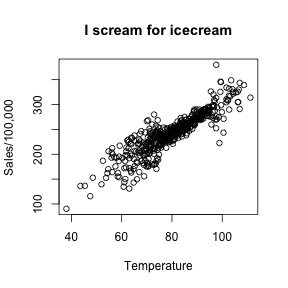Here we definitely see a relationship between the temperature in a city and the sales of icecream. However, we notice that our errors are not exactly homoskedastic. We see that for both lower temperatures and very high temperatures, the variance is larger than the middle temperatures. So we will have to deal with that.

Let's start by running a linear regression using the lm() function and summarizing the results:

fit1 <- lm(sales ~ temp + female, data = icecream)
summary(fit1)

##
## Call:
## lm(formula = sales ~ temp + female, data = icecream)
##
## Residuals:
##    Min     1Q Median     3Q    Max
## -76.34  -8.02   0.34   8.19  84.97
##
## Coefficients:
##             Estimate Std. Error t value Pr(>|t|)
## (Intercept)  23.9164    41.1872    0.58     0.56
## temp          2.9716     0.0671   44.30   <2e-16 ***
## female      -37.8194    81.0642   -0.47     0.64
## ---
## Signif. codes:  0 '***' 0.001 '**' 0.01 '*' 0.05 '.' 0.1 ' ' 1
##
## Residual standard error: 18.1 on 497 degrees of freedom
## Multiple R-squared:  0.799,  Adjusted R-squared:  0.798
## F-statistic:  985 on 2 and 497 DF,  p-value: <2e-16

names(summary(fit1))

##   "call"          "terms"         "residuals"     "coefficients"  "aliased"       "sigma"         "df"            "r.squared"     "adj.r.squared"
##  "fstatistic"    "cov.unscaled"


We see the strong association between temperature and icecream sales, but no relationship between the gender ratio and icecream. Don't forget that you can extract many things from the summary of the lm object (read up on extracting information using names() in this post.) Now let's deal with the heteroskedasticity. We know that under the constant variance assumption,

$V[\hat\beta] = (X'X)^{-1}X'\Sigma X(X'X)^{-1}$

where

$\Sigma = \sigma^2I$

and we can find an unbiased estimate of $$\sigma^2$$ by summing the squared the residuals. However, in this case, we see that the variance is not constant, so the naive covariance of the OLS estimator is biased. To fix this we employ the White Huber, or sandwich, method of robust standard errors. The sandwich method assumes that

$\Sigma = diag(\sigma_1^2, ... , \sigma_n^2)$

and again the $$\hat\sigma_i^2$$ are estimated via the residuals $$\hat u_i^2$$. In R, we can use the sandwich package to estimate robust standard errors this way:

library(sandwich)
cov.fit1 <- vcovHC(fit1, type = "HC")
rob.std.err <- sqrt(diag(cov.fit1))


The vcovHC() function returns the variance-covariance matrix under the assumption of “HC” (Heteroskedasticity-consistent) estimation. We then take the diagonal of this matrix and square root it to calculate the robust standard errors. You could do this in one line of course, without creating the cov.fit1 object.

Now, we can put the estimates, the naive standard errors, and the robust standard errors together in a nice little table. We save the naive standard errors into a vector and then column bind the three columns together like so:

naive.std.err<-summary(fit1)coefficients[,2] estimate.table <- cbind("Estimate" = coef(fit1), "Naive SE"= naive.std.err, "Robust SE" = rob.std.err) estimate.table  ## Estimate Naive SE Robust SE ## (Intercept) 23.916 41.18715 40.40784 ## temp 2.972 0.06707 0.08379 ## female -37.819 81.06423 78.92591  That's a good start. But of course we want p-values and, even better, upper and lower 95% confidence intervals, so we can add those to the table: better.table <- cbind("Estimate" = coef(fit1), "Naive SE" = naive.std.err, "Pr(>|z|)" = 2 * pt(abs(coef(fit1)/naive.std.err), df=nrow(icecream)-2, lower.tail = FALSE), "LL" = coef(fit1) - 1.96 * naive.std.err, "UL" = coef(fit1) + 1.96 * naive.std.err, "Robust SE" = rob.std.err, "Pr(>|z|)" = 2 * pt(abs(coef(fit1)/rob.std.err), df=nrow(icecream)-2, lower.tail = FALSE), "LL" = coef(fit1) - 1.96 * rob.std.err, "UL" = coef(fit1) + 1.96 * rob.std.err) rownames(better.table)<-c("Constant", "Temperature", "Gender Ratio") better.table  ## Estimate Naive SE Pr(>|z|) LL UL Robust SE Pr(>|z|) LL UL ## Constant 23.916 41.18715 5.617e-01 -56.81 104.643 40.40784 5.542e-01 -55.283 103.116 ## Temperature 2.972 0.06707 6.750e-175 2.84 3.103 0.08379 2.324e-138 2.807 3.136 ## Gender Ratio -37.819 81.06423 6.410e-01 -196.71 121.066 78.92591 6.320e-01 -192.514 116.875  A bit wide, but there we are. We are finished with our linear regression analysis. But now we would like to export this into a pdf or word document so we can send it to our advisor who will be very excited about this cutting edge icecream research. There are two options that I'll go over, xtable() and stargazer(). ### xtable The first option is xtable. You can put in just about any object (dataframe, table, matrix, lm, glm, etc) and it will create latex code for you that you can copy and paste into latex. This is how the resulting table will look in latex: xtable(fit1)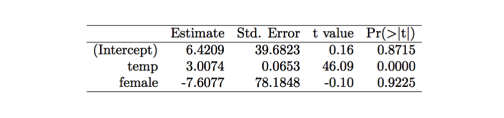But now what we really want is to compare the naive to the robust in one table. If we want to use xtable, we will have to put those vectors together ourselves, the way we have done above. You can't input multiple models into the xtable function. xtable(better.table, digits = 3, caption = "Comparison of Naive and Robust SEs")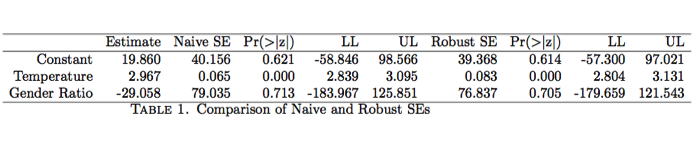This table is not all that attractive though - it's too wide for the amount of information it's imparting and it's not how we are used to seeing regression results. We can move on to another way of exporting tables that is better. ### stargazer The package I encourage you to try is called stargazer. It can make the task of creating tables and reporting results easier and more attractive. The basic function is just stargazer(fit1), which prints out latex output into the R console. You can copy the latex code and paste it into a latex document. Here is the output for the basic stargazer table produced just from the model itself with all of the function defaults: library(stargazer) stargazer(fit1)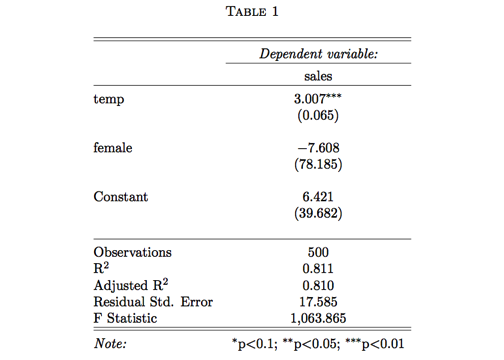If you want a text file instead to put into a word document, there is an option for that, stargazer(fit1, type = "text", out = "reg_results.txt")  and it will export a text file of the same table. This is a nice start, but the great part about this function is that if we have multiple models like we do with our icecream example, we can juxtapose them without having to create a table ourselves using cbind. We just put in as many model objects as we want and it will create that many columns. Then we can adjust the standard errors of the models. Finally, we can change a lot of the physical appearance with stargazer's many customizable options. Here is the code: stargazer(fit1, fit1, se = list(naive.std.err,rob.std.err), title="Regression Results", align=TRUE, dep.var.labels=c("Icecream sales"), covariate.labels=c("Temperature","Gender ratio"), no.space=TRUE, omit.stat=c("LL","ser","f", "rsq"), column.labels=c("Naive SE", "Robust SE"), dep.var.caption="", model.numbers=FALSE)  This is a lot of code in one bit but all of the options are very self-explanatory (there's also descriptions of every option in the help file). So in this table we are going to: • Juxtapose two models - the linear model with naive SEs and the linear model with robust SEs - so we put in fit1, fit1 which takes the same coefficients for both columns of the table. • Then specify the two different standard errors which we list together: se=list(naive.std.err, rob.std.err). This is not necessary if you just go with the model produced standard errors. • Then we add a title for the whole thing • Add a dependent variable label and some covariate labels • Remove all the spaces • Take out some of the default stats that show up • Label the columns • Take out the dependent variable caption • and take out the model numbers.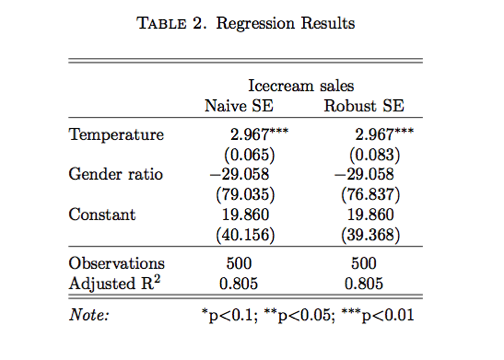Looks pretty great! So there you go, in this way you can conduct linear regression with robust standard errors and then report your results in a physically attractive and easy way. library(sandwich) library(stargazer) library(pander) library(xtable) options(width=150) Motivation Basic linear regression in R is super easy. Just use the lm() function, summarize the model with summary() and you're done. But as researchers we need more than that. What if our model is more complicated, like if our errors are heteroskedastic? And once we have run our regressions with our adjusted standard errors, how do we export the results for presentation in word or latex documents? This post will go over exactly these things, with the help of the stargazer package created by my fellow Harvard grad student Marek Hlavac. More posts about stargazer can be found [here on this blog.](http://www.r-statistics.com/2013/07/tailor-your-tables-with-stargazer-new-features-for-latex-and-text-output/) Today we are going to analyze how temperature affects ice cream sales. We just make ourselves a little dataset like so: set.seed(5) temp <- rnorm(500, mean=80, sd=12) sales <- 2+temp*3 for(i in 1:length(sales)){ if(temp[i]<75 | temp[i]>95) sales[i] <- sales[i]+rnorm(1,0,25 ) else sales[i] <- sales[i]+rnorm(1,0,8 ) } female <- rnorm(500, mean=.5, sd=.01) icecream <- as.data.frame(cbind(temp, sales, female)) head(icecream) So there we have the temperature of 500 cities, sales per 100,000 people, and gender ratio of each of these cities. Let's plot out the temperature and sales. plot(icecreamtemp, icecream$sales, xlab="Temperature", ylab="Sales/100,000", main="I scream for icecream") Here we definitely see a relationship between the temperature in a city and the sales of icecream. However, we notice that our errors are not exactly homoskedastic. We see that for both lower temperatures and very high temperatures, the variance is larger than the middle temperatures. So we will have to deal with that. Let's start by running a linear regression using the lm() function and summarizing the results: fit1<-lm(sales~temp+female, data=icecream) summary(fit1) names(summary(fit1)) We see the strong association between temperature and icecream sales, but no relationship between the gender ratio and icecream. Don't forget that you can extract many things from the summary of the lm object (read up on extracting information using names() in [this post.](http://rforpublichealth.blogspot.com/2013/03/extracting-information-from-objects.html)) Now let's deal with the heteroskedasticity. We know that under the constant variance assumption, $$V[\hat\beta] = (X'X)^{-1}X'\Sigma X(X'X)^{-1}$$ where $$\Sigma = \sigma^2I$$ and we can find an unbiased estimate of$\sigma^2$by summing the squared the residuals. However, in this case, we see that the variance is not constant, so the naive covariance of the OLS estimator is biased. To fix this we employ the White Huber, or sandwich, method of robust standard errors. The sandwich method assumes that $$\Sigma = diag(\sigma_1^2, ... , \sigma_n^2)$$ and again the$\hat\sigma_i^2$are estimated via the residuals$\hat u_i^2$. In R, we can use the sandwich package to estimate robust standard errors this way: library(sandwich) cov.fit1 <- vcovHC(fit1, type="HC") rob.std.err <- sqrt(diag(cov.fit1)) The vcovHC() function returns the variance-covariance matrix under the assumption of "HC" (Heteroskedasticity-consistent) estimation. We then take the diagonal of this matrix and square root it to calculate the robust standard errors. You could do this in one line of course, without creating the cov.fit1 object. Now, we can put the estimates, the naive standard errors, and the robust standard errors together in a nice little table. We save the naive standard errors into a vector and then column bind the three columns together like so: naive.std.err<-summary(fit1)$coefficients[,2] estimate.table <- cbind("Estimate" = coef(fit1), "Naive SE"= naive.std.err, "Robust SE" = rob.std.err) estimate.table That's a good start. But of course we want p-values and, even better, upper and lower 95% confidence intervals, so we can add those to the table: better.table <- cbind("Estimate" = coef(fit1), "Naive SE" = naive.std.err, "Pr(>|z|)" = 2 * pt(abs(coef(fit1)/naive.std.err), df=nrow(icecream)-2, lower.tail = FALSE), "LL" = coef(fit1) - 1.96 * naive.std.err, "UL" = coef(fit1) + 1.96 * naive.std.err, "Robust SE" = rob.std.err, "Pr(>|z|)" = 2 * pt(abs(coef(fit1)/rob.std.err), df=nrow(icecream)-2, lower.tail = FALSE), "LL" = coef(fit1) - 1.96 * rob.std.err, "UL" = coef(fit1) + 1.96 * rob.std.err) rownames(better.table)<-c("Constant", "Temperature", "Gender Ratio") better.table A bit wide, but there we are. We are finished with our linear regression analysis. But now we would like to export this into a pdf or word document so we can send it to our advisor who will be very excited about this cutting edge icecream research. There are two options that I'll go over, xtable() and stargazer(). xtable print(xtable(fit1), type="html") The first option is xtable. You can put in just about any object (dataframe, table, matrix, lm, glm, etc) and it will create latex code for you that you can copy and paste into latex. This is how the resulting table will look in latex: xtable(fit1) library(png) library(grid) img <- readPNG("figure/basicxtable.png") grid.raster(img) But now what we really want is to compare the naive to the robust in one table. If we want to use xtable, we will have to put those vectors together ourselves, the way we have done above. You can't input multiple models into the xtable function. xtable(better.table, digits=3, caption="Comparison of Naive and Robust SEs") library(png) library(grid) img <- readPNG("figure/xtable.png") grid.raster(img) This table is not all that attractive though - it's too wide for the amount of information it's imparting and it's not how we are used to seeing regression results. We can move on to another way of exporting tables that is better. stargazer The package I encourage you to try is called stargazer. It can make the task of creating tables and reporting results easier and more attractive. The basic function is just stargazer(fit1), which prints out latex output into the R console. You can copy the latex code and paste it into a latex document. Here is the output for the basic stargazer table produced just from the model itself with all of the function defaults: library(stargazer) stargazer(fit1) library(png) library(grid) img <- readPNG("figure/basic_sg.png") grid.raster(img) If you want a text file instead to put into a word document, there is an option for that, stargazer(fit1, type="text", out="reg_results.txt") and it will export a text file of the same table. This is a nice start, but the great part about this function is that if we have multiple models like we do with our icecream example, we can juxtapose them without having to create a table ourselves using cbind. We just put in as many model objects as we want and it will create that many columns. Then we can adjust the standard errors of the models. Finally, we can change a lot of the physical appearance with stargazer's many customizable options. Here is the code: stargazer(fit1, fit1, se = list(naive.std.err,rob.std.err), title="Regression Results", align=TRUE, dep.var.labels=c("Icecream sales"), covariate.labels=c("Temperature","Gender ratio"), no.space=TRUE, omit.stat=c("LL","ser","f", "rsq"), column.labels=c("Naive SE", "Robust SE"), dep.var.caption="", model.numbers=FALSE) This is a lot of code in one bit but all of the options are very self-explanatory (there's also descriptions of every option in the help file). So in this table we are going to: * Juxtapose two models - the linear model with naive SEs and the linear model with robust SEs - so we put in fit1, fit1 which takes the same coefficients for both columns of the table. * Then specify the two different standard errors which we list together: se=list(naive.std.err, rob.std.err). This is not necessary if you just go with the model produced standard errors. * Then we add a title for the whole thing * Add a dependent variable label and some covariate labels * Remove all the spaces * Take out some of the default stats that show up * Label the columns * Take out the dependent variable caption * and take out the model numbers. library(png) library(grid) img <- readPNG("figure/sg33a.png") grid.raster(img) Looks pretty great! So there you go, in this way you can conduct linear regression with robust standard errors and then report your results in a physically attractive and easy way.

#### 14 comments:

1.Isn't it dangerous to write,
ifelse(temp < 75, rnorm(500, mean = 0, sd = 25), ifelse(temp > 95, rnorm(500, mean = 0, sd = 25), rnorm(500, mean = 0, sd = 8)))

1.Yeah you're right, that was a little weird. I changed it, thanks.

2.I assume you made this post in knitr. I am trying to convert a knitr html to blogger post but am having trouble with the latex to display. Any advice?

1.Yeah well I do it in this very hacky way where I just save the html file in a public folder and then I refer to it in an iframe statement. Not great, if you find a better way, let me know.

3.Very cool. I've used outreg (part of the rockchalk package) for output in the past. Have you used it and have any opinions on how it compares to stargazer?
One frustrating thing about our field is that although LaTex is clearly superior in lots of ways for article formatting, many of the journals just want you to submit everything in Word. Stata has a really great package, xml_tab, to output nicely formatted tables to an Excel file which are then easy to paste into Word. I wonder if there's anything similar for R?

1.I haven't used outreg, I'll take a look. I agree about the word/latex journal situation. With stargazer you can export as ASCII text, which you can cut and paste into word or paste into excel and then fix the formatting on it which adds an extra step. I don't know of any direct way to export to excel.

There are a bunch of programs that convert from pdf to word. Pandoc is one program that I will test out soon - I'll let you know if the results are good.
http://johnmacfarlane.net/pandoc/demos.html

4.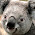In addition to the options you suggested another useful library on the latex side for tables is pgfplotstable. I tend to run similar analyses where I have preformatted the latex document's table. With pgfplotstable I can directly pull in a text file generated in R and have it format itself, so no copy and pasting. Its really handy if I adjust things, all I have to do is tell latex to rebuild the document and the table is done for me.

1.Great, thanks I will check it out.

5.Hi, thanks for the post. I have a problem. If I save the output type="latex" and out ="test.tex" than the output includes automatically \documentclass{article} and begin{document} into the file. Therefore I cannot use the \input command in latex. Do you have any suggestions how to surpress that stargazer includes automatically latex preemble commands? Thanks!

1.I talked to the author of the package, who's a friend of mine, and he says the following:

"The current version does not have an argument that would remove these lines from .tex output, but the feature will be included in the next release (hopefully soon). One thing you could nevertheless do right now is to save stargazer output as a vector of strings into an object, and then subset to only include the lines you're interested in. If you
have any further questions or comments, please do not hesitate to e-mail the package author directly."

Here is contact information: http://cran.r-project.org/web/packages/stargazer/index.html

6.Your dazzling work has won my heart. I’ll come soon to your site with new hope.
mango exporter pakistan

7.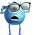According to the showed database it can be seen as the value of Exporting in the economic Growth.

8.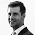heelo.
i used this :library(stargazer)
stargazer(fit1)

but the result is printed in the console :
% Table created by stargazer v.5.2 by Marek Hlavac, Harvard University. E-mail: hlavac at fas.harvard.edu
% Date and time: Mon, Nov 30, 2015 - 10:01:57 PM
\begin{table}[!htbp] \centering
\caption{}
\label{}
\begin{tabular}{@{\extracolsep{5pt}}lc}
\\[-1.8ex]\hline
etc

what i should change?

1.yes, the result is latex code that you copy and paste into latex. You can download latex for free if you google it.

Note: Only a member of this blog may post a comment.